# Introduction

This is my “best paper” of the CVPR 2019 edition. It revisits the notion of robust regression loss function $$\rho(.)$$,

$\arg \min_\theta \rho \left( f_\theta(x)-y \right )$

as it proposes a new general loss function which is a superset of many existing losses. The parameters of this loss can be automatically adapted to fit the true nature of the problem.

# Methods

$$\rho(.)$$ can be an L2 or an L1 function. However, it can also be a robust function like a Welsch loss, a Geman-McClure loss, or a Cauchy loss. The author shows that all these functions are special cases of a more general fobust function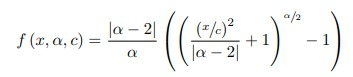where $$\alpha$$ is a shape parameter. By playing with $$\alpha$$, one can recover the various loss functions mentioned before.

Although $$\alpha$$ can be seen as an extra hyperparameter that needs to be tuned, one can define it as a trainable parameter. To do so, the author encapsulates the loss into a PDF formulation: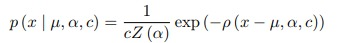where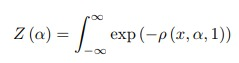so the optimization function becomes

$\arg \min_{\theta,\alpha} -log(p(x|\alpha) = \rho \left( x,\alpha \right ) + log(Z(\alpha))$

where $$log(Z(\alpha))$$ is some complicated analytical function which the author approximates with a cubic spline function.

Having $$Z(.)$$ in the loss function is important because without it, the system would trivially minimize the cost of outliers by setting $$\alpha$$ to be as small as possible. However, as shown in Fig.2, reducing $$\alpha$$ will decrease the loss of outlisers but will increase the loss of inliers.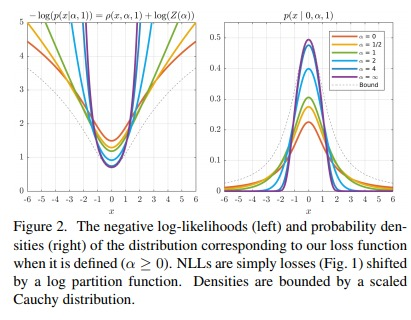# Results

Results are quite convincing on various applications like VAEs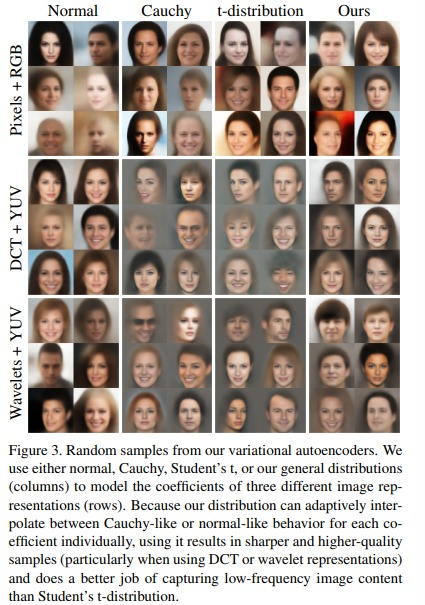and monocular depth estimation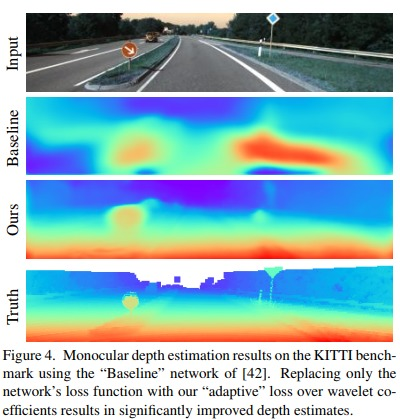# Video

The author recorded a nice video. Don’t miss it!